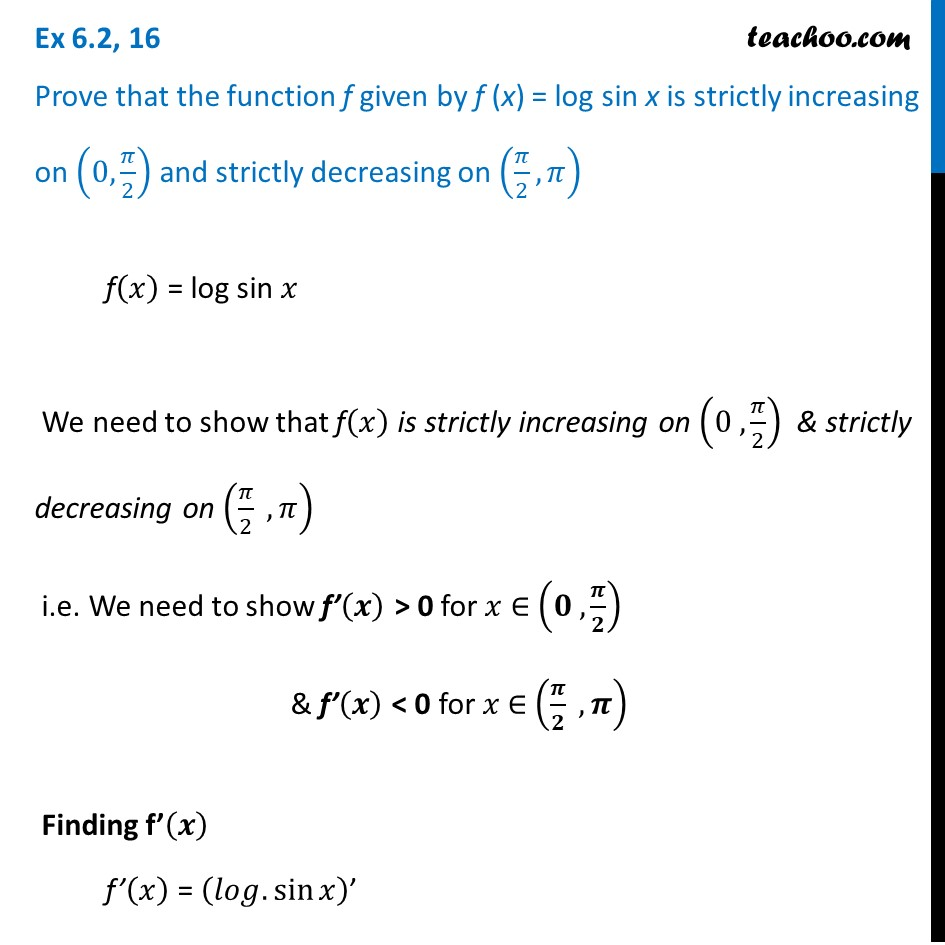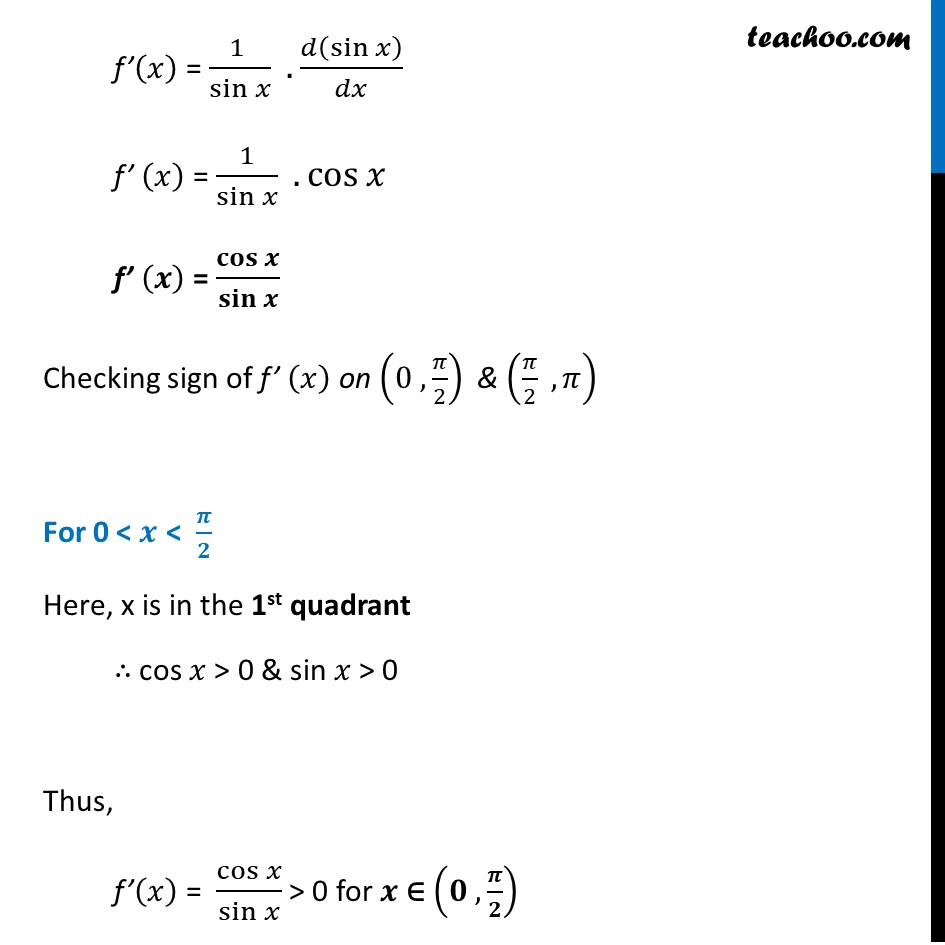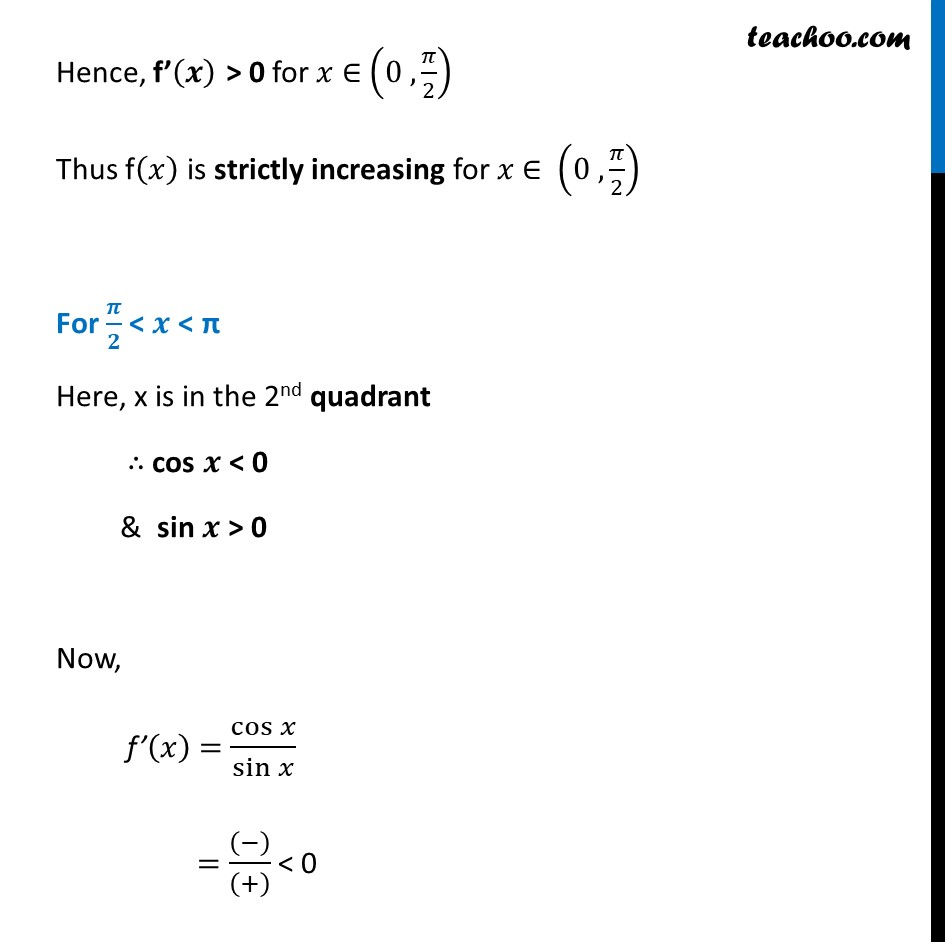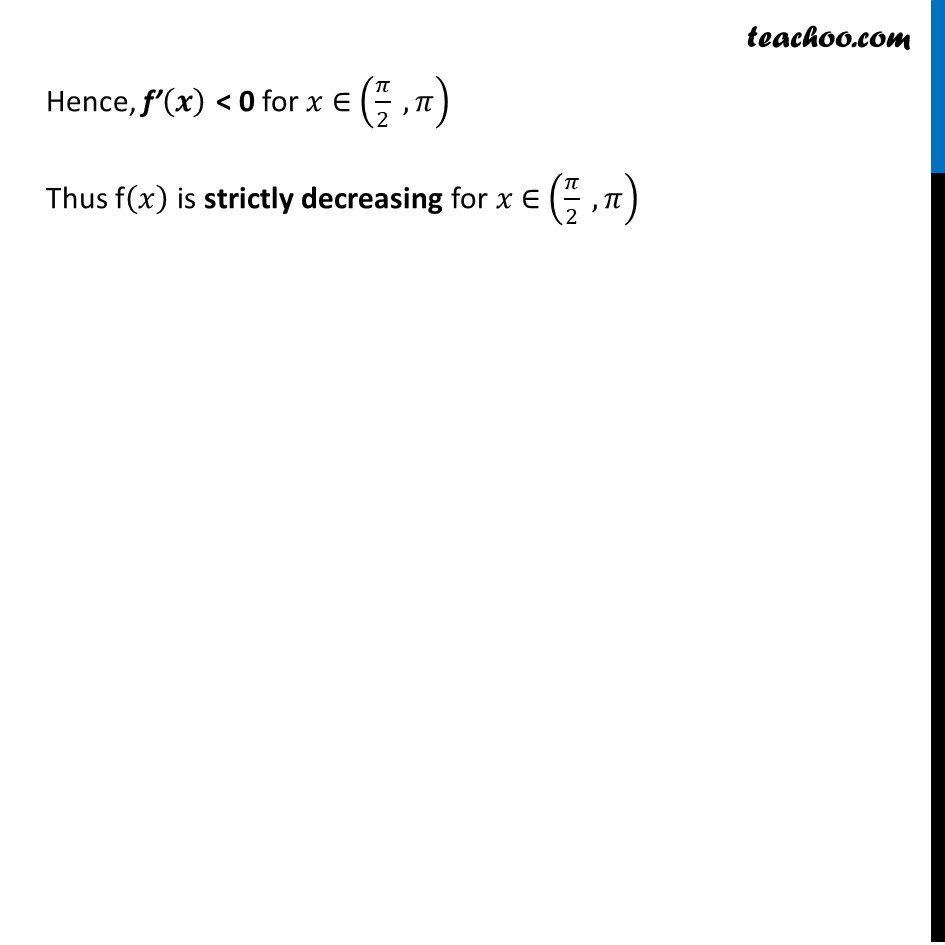Ex 6.2

Chapter 6 Class 12 Application of Derivatives
Serial order wiseLearn in your speed, with individual attention - Teachoo Maths 1-on-1 Class

### Transcript

Ex 6.2, 16 Prove that the function f given by f (x) = log sin x is strictly increasing on (0,𝜋/2) and strictly decreasing on (𝜋/2,𝜋) f(𝑥) = log sin 𝑥 We need to show that f(𝑥) is strictly increasing on (0 , 𝜋/2) & strictly decreasing on (𝜋/2 , 𝜋) i.e. We need to show f’(𝒙) > 0 for 𝑥 ∈ (𝟎 , 𝝅/𝟐) & f’(𝒙) < 0 for 𝑥 ∈ (𝝅/𝟐 , 𝝅) Finding f’(𝒙) f’(𝑥) = (𝑙𝑜𝑔.sin⁡𝑥 )’ f’(𝑥) = (1 )/sin⁡𝑥 . 𝑑(sin⁡𝑥 )/𝑑𝑥 f’ (𝑥) = 1/sin⁡𝑥 .cos⁡𝑥 f’ (𝒙) = 𝐜𝐨𝐬⁡𝒙/𝐬𝐢𝐧⁡𝒙 Checking sign of f’ (𝑥) on (0 , 𝜋/2) & (𝜋/2 , 𝜋) For 0 < 𝒙 < 𝝅/𝟐 Here, x is in the 1st quadrant ∴ cos 𝑥 > 0 & sin 𝑥 > 0 Thus, f’(𝑥) = cos⁡𝑥/sin⁡𝑥 > 0 for 𝒙 ∈ (𝟎 , 𝝅/𝟐) Hence, f’(𝒙) > 0 for 𝑥 ∈ (0 , 𝜋/2) Thus f(𝑥) is strictly increasing for 𝑥 ∈ (0 , 𝜋/2) For 𝝅/𝟐 < 𝒙 < π Here, x is in the 2nd quadrant ∴ cos 𝒙 < 0 & sin 𝒙 > 0 Now, f’(𝑥) = cos⁡𝑥/sin⁡𝑥 = ((−))/((+) ) < 0 Hence, f’(𝒙) < 0 for 𝑥 ∈ (𝜋/2 , 𝜋) Thus f(𝑥) is strictly decreasing for 𝑥 ∈ (𝜋/2 , 𝜋)